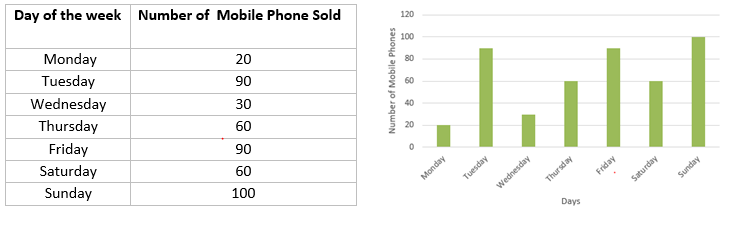# Data Handling Notes

## Data collection and Orgainisation

• The information collected in the form of numbers is called Data.
• Data is collected to obtain usefull and insightful information about the material
• Data  needs to be organised in a proper table, so that it becomes easy to understand and interpret.

## Range

The difference between the highest and lowest observations in a given data is called its Range

## Mean or Arithmetic Mean

• Average is a number that represents or shows the central tendency of a group of observations or data.
• Arithmetic mean is one of the representative values of data.
• The average or Arithmetic Mean (A.M.) or simply mean is defined as follows:
$mean = \frac {\text{Sum of all observations}}{\text {number of observations}}$

1. What is the mean of 26, 28, 25, 32, 24
Solution
$Mean =\frac { 26 + 28 + 25 + 32 + 24}{5} =27$
2. The mean of 10 observations was calculated as 40. It was detected on rechecking that the value of 45 was wrongly copied as 15. Find the correct mean
Solution
$mean = \frac {\text{Sum of all observations}}{\text {number of observations}}$
or
$40 = \frac {\text{Sum of all observations}}{10}$
So, sum of all observations = 400
But this is incorrect sum, since one observation was copied wrongly.
So, correct sum = Incorrect sum � Incorrect observation + correct observation
= 400 � 15 + 45
= 430
$\text{Correct Mean} = \frac {\text {Correct Sum Number of observations}}{10}$
$=\frac {430}{10} =43$

## What is Mode

Mode is another form of central tendency or representative value. The mode of a set of observations is the observation that occurs most often.
1. Find the mode of the numbers: 9, 3, 2, 2, 3, 4, 5, 5, 5, 6, 6, 8,2,10
Solution
First we need to arrange them in ascending order 2, 2, 2, 3, 3, 4, 5, 5, 5, 6, 6, 8,9,10 Here, 2 and 5 both occur three times. Therefore, they both are modes of the data

2. Find the mode of the numbers: 1, 4, 2, 2, 4, 4, 1, 3, 7, 8, 9, 9,1,2,5,10,4
Solution
First we need to arrange them in ascending order 1,1,1,2,2,2,3,4,4,4,4,5,,7,8,9,9,10 Mode is 4 as it occurs maximum times

## What is Median

Median is also a form of representative value. It refers to the value which lies in the middle of the data with half of the observations above it and the other half below it.
For Odd Number of Observation
Median = Value of $\frac {1}{2} (n+1)}$ observation

For even Number of Observation
Median = $\frac {1}{2}$ {$\frac {1}{2}n$th observation + $(\frac {n}{2} + 1)$th observation}

1. Find the median of 106, 110, 123, 125, 117, 120, 112, 115, 110, 120, 115, 102, 115, 115, 109, 115, 101
Solution
First we need to arrange them in ascending order
101,102,106,109,110,110,112,115,115,115,115,115,117,120,120,123,125
Number of observation is 17. So medain will 9 terms
So, Median=115

2. Find the median of the data: 24, 36, 46, 17, 18, 25, 35
Solution
First we need to arrange them in ascending order
17, 18, 24, 25, 35, 36, 46
Median is the middle observation. Therefore 25 is the median.

## Bar Graph

A bar graph is a representation of numbers using bars of uniform widths.## Double bar graphs

It help to compare two collections of data at a glance.

## Probability or Chance

When you toss the coin, Head or tail may come. You can not predict whether head or tail will occur. But There is a chance that head may happened.So The situation that may or may not happen, have a chance of happening.
Now chance is measure by Probability.
Probability is defined as
$\text {probability of an event} = \frac {\text {Number of outcomes favourable to the event}}{ \text{Total number of outcomes in the experiment}}$
Important Note
1. The probablity of an event which is certain to happen is ‘1’.
2. The probability of an event which is impossible to happen is ‘0’.

We have another way to defined to probability also which is called emprical probability
\$P(E ) = \frac {\text {Number of Trials in which E occurs}}{\text {Total Number of trials}}